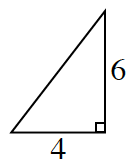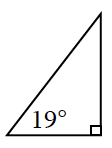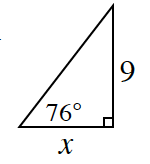### Home > INT2 > Chapter 3 > Lesson 3.2.3 > Problem3-94

3-94.

1. Thalia did not have a tool to help her determine the slope angle $θ$ in the triangle at right. However, she claims that the slope angle has to be more than $45^\circ$. Do you agree with Thalia? Why?

An isosceles right triangle has a slope of $1$ and slope angle of $45^\circ$. Does this triangle have a larger or smaller ratio? What does this tell us about the slope angle?

Yes, because the slope ratio is greater than $1$,the angle must be greater than $45^\circ$.1. Lyra was trying to determine the slope ratio for the triangle at right, and she says the answer is $\frac { \Delta y } { \Delta x }= 2.675$. Isiah claims that cannot be correct. Who is right? How do you know?

Use the same method as in part (a).1. Without determining the actual value, what information do you know about $x$ in the diagram at right?

If the slope angle is $76^\circ$, is the slope ratio more than $1$ or less than $1$?
Does this mean that $x$ is greater than $9$ or less than $9$?